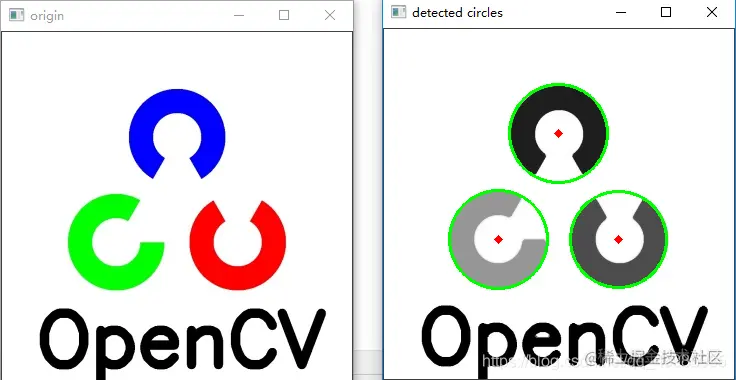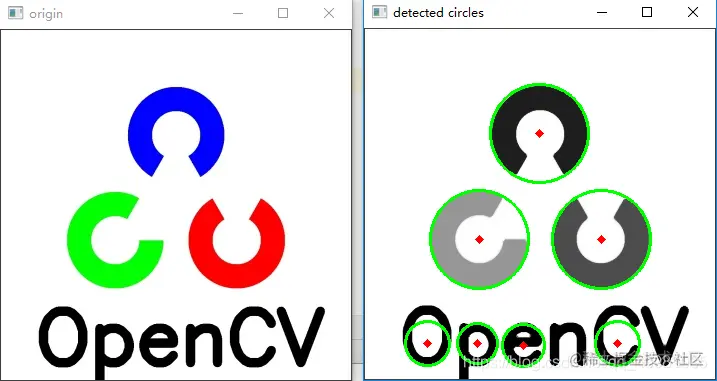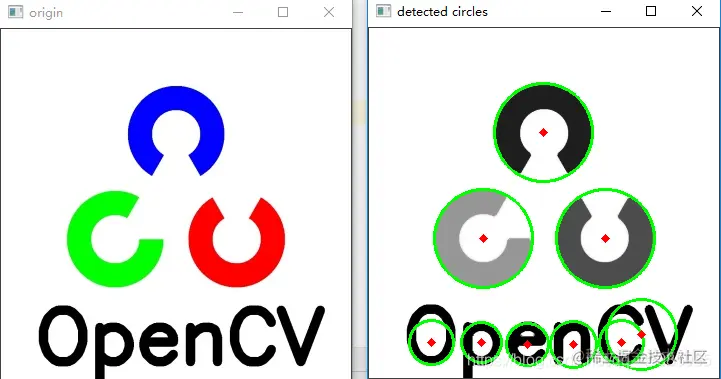# Python, Hough circle transformation in opencv

2022-01-30 17:12:50

# Python,OpenCV Hoff circle transformation in

This blog will learn how to use Hoff circle transform to find circles in images ,OpenCV Use cv2.HoughCircles() Realize Hough circle transformation .

circles = cv2.HoughCircles(img, cv2.HOUGH_GRADIENT, 1, 20, param1=50, param2=40, minRadius=25, maxRadius=0)

• img: Gray scale image to be detected
• cv2.HOUGH_GRADIENT： The method of detection , Hough gradient
• 1： The detected circle has the same size as the original image ,dp=2, The detected circle is half of the original image
• 20： The minimum distance between the centers of adjacent circles detected （ If the parameter is too small , Except for a real circle , It is also possible to erroneously detect multiple adjacent circles . If it is too big , Some circles may be missed .）
• param1： stay #HOUGHŠu In the case of gradients , It's higher . Two thresholds are passed to Canny Edge detector （ The lower one is twice as small ）.
• param2： stay #HOUGHŠu In the case of gradients , It is the accumulator threshold of the center of the circle in the detection stage . The smaller it is , The more likely it is to detect false circles ;
• maxRadius： Maximum circle radius , If <=0, The maximum image size is used . If <0, The center of the radius is not found .

## 1. design sketch

The original picture VS The effect drawing of detection circle is as follows ：

As shown in the right figure below 3 An outer round green , The center red is successfully detected ;The minimum radius of the circle is set by 25 Adjusted for 10, The circle may also be detected incorrectly , The renderings are as follows ：Threshold value for detecting circle radius （param2 Set up 35） Result , Minimum circle radius setting 10, The circle may also be detected incorrectly , The renderings are as follows ：## 2. Source code

``````#  Hoff circle test

import cv2
import numpy as np

cv2.imshow("origin", cimg)
cv2.waitKey(0)
img = cv2.cvtColor(cimg,cv2.COLOR_BGR2GRAY)
img = cv2.medianBlur(img, 5)
cimg = cv2.cvtColor(img, cv2.COLOR_GRAY2BGR)

# - img:  Gray scale image to be detected
# - cv2.HOUGH_GRADIENT： The method of detection , Hough gradient
# - 1： The detected circle has the same size as the original image ,dp=2, The detected circle is half of the original image
# - 20： The minimum distance from the center of the detected circle （ If the parameter is too small , Except for a real circle , It is also possible to erroneously detect multiple adjacent circles . If it is too big , Some circles may be missed .）
# - param1： stay #HOUGHŠu In the case of gradients , It's higher .  Two thresholds are passed to Canny Edge detector （ The lower one is twice as small ）.
# - param2： stay #HOUGHŠu In the case of gradients , It is the accumulator threshold of the center of the circle in the detection stage . The smaller it is , The more likely it is to detect false circles ;
# - minRadius： Minimum circle radius , False circles may also be detected
# - maxRadius： Maximum circle radius , If <=0, The maximum image size is used . If <0, The center of the radius is not found .
circles = cv2.HoughCircles(img, cv2.HOUGH_GRADIENT, 1, 20,
#  The minimum circle radius setting is not appropriate , False circles may also be detected
# circles = cv2.HoughCircles(img, cv2.HOUGH_GRADIENT, 1, 20,
# circles = cv2.HoughCircles(img, cv2.HOUGH_GRADIENT, 1, 20,

circles = np.uint16(np.around(circles))

print(len(circles))
print(circles)
for i in circles[0, :]:
#  Draw outer circle （ Blue ）
cv2.circle(cimg, (i, i), i, (0, 255, 0), 2)

#  Draw the center of the circle （ Red ）
cv2.circle(cimg, (i, i), 2, (0, 0, 255), 3)

cv2.imshow('detected circles', cimg)
cv2.waitKey(0)
cv2.destroyAllWindows()
Copy code ``````